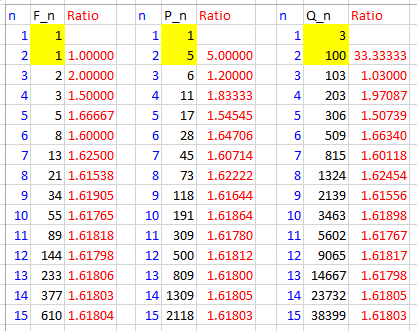# Generalizing and Summing the Fibonacci Sequence

Continuing our look at the Fibonacci sequence, we’ll extend the idea to “generalized Fibonacci sequences” (with different starting numbers), and see that the ratio of consecutive terms is the same in general as in the usual special case. Then we’ll look at the sum of terms of both the special and general sequence, turning it into a series. This turns out to be as simple as you could imagine!

## Generalized Fibonacci sequences

Recall that the Fibonacci sequence is defined by specifying the first two terms as $$F_1=1$$ and $$F_2=1$$, together with the recursion formula $$F_{n+1}=F_n+F_{n-1}$$. We have seen how to use this definition in various kinds of proofs, and also how to find an explicit formula for the nth term, and that the ratio between successive terms approaches the golden ratio, $$\phi$$, in the limit.

Similar questions can be raised about any sequence that has the same recursion as the Fibonacci sequence, but starts with a different pair of numbers. That is the subject of a question from 2002:

Generalised 'Fibonacci' Series and Phi

I have searched but I can't find an answer to this.

I have shown with a spreadsheet that a Fibonacci-style series that starts with any two numbers at all, and adds successive items, produces a ratio of successive items that converges to phi in about the same number of terms as for the 1 1 2 3 5 etc. basic Fibonacci series.  I can't find reference to this - maybe I don't know how to look - surely this is well known?  Is it - and is it provable?

None of these series converges to $$\phi$$ in a finite number of terms, but presumably Stuart means that it converges to about the same precision in about the same number of steps. Here is an example of a spreadsheet showing this (which is very easy to make):The first column is Fibonacci proper, starting with 1, 1; these are the numbers we call the Fibonacci numbers. The second starts with 1, 5 (which we’ll be seeing later), and the third, just to be very different, starts with 3, 100. The ratios in each case rapidly converge toward 1.618034…, which is $$\phi$$.

Hi, Stuart,

To prove your conjecture we will delve into formulas of generalized Fibonacci sequences (sequences satisfying X(n) = X(n-1) + X(n-2) ). Let's first investigate two very special members of the family of generalized Fibonacci sequences, those of the form

X(1)=g=g^1, X(2)=g^2, X(3)=g^3, ..., X(n)=g^n  [g nonzero]

In other words, is it possible for a sequence with this recursion to also be a geometric sequence? That’s not the first question I’d think to ask about generalized Fibonacci sequences;  but it turns out to be interesting:

Since X(n)=X(n-1)+X(n-2), we must have:

g^n = g^(n-1) + g(n-2)
g^n - g^(n-1) - g(n-2) = 0
g^(n-2) * (g^2 - g - 1) = 0
g^2 - g - 1 = 0   [we can divide by g^(n-2) because g is nonzero]

So g must be one of the roots of g^2 - g - 1 = 0, so it must be one of the following numbers:

Phi = (1+SQRT(5))/2  (the Golden Ratio)
1-Phi = (1-SQRT(5))/2

Only these two numbers can work as the common ratio. We could actually start the sequence with any number we like (including 1), so there are many such geometric “Fibonacci” sequences (which would be multiples of these); but taking the sequences as $$A_n = \phi^n$$ and $$B_n = (1-\phi)^n$$ will keep the formulas simple.

So we have two very special generalized Fibonacci sequences:

Phi^1, Phi^2, Phi^3, ..., Phi^n, ...
(1-Phi)^1, (1-Phi)^2, ..., (1-Phi)^n, ...

I leave it for you to check that, when you have two generalized Fibonacci sequences A(n) and B(n), and two numbers a and b, then a*A(n) + b*B(n) is a generalized Fibonacci sequence.

Let’s do that: If $$A_n$$ and $$B_n$$ satisfy the recursions $$A_n=A_{n-1}+A_{n-2}$$ and $$B_n=B_{n-1}+B_{n-2}$$, and $$C_n=aA_n+bB_n$$, then $$C_{n-1}+C_{n-2} = (aA_{n-1}+bB_{n-1})+(aA_{n-2}+bB_{n-2})\\ = a(A_{n-1}+A_{n-2})+b(B_{n-1}+B_{n-2}) = aA_n+bB_n = C_n.$$

So any linear combination of any two generalized Fibonacci sequences is also a generalized Fibonacci sequence.

In fact, all generalized Fibonacci sequences can be calculated in this way from Phi^n and (1-Phi)^n. This can be seen from the fact that any two initial terms can be created by some a and b from two (independent) pairs of initial terms from A(n) and B(n), and thus also from Phi^n and (1-Phi)^n.

So each generalized Fibonacci sequence can be written as

X(n) = a*Phi^n + b*(1-Phi)^n.

### 1 thought on “Generalizing and Summing the Fibonacci Sequence”

This site uses Akismet to reduce spam. Learn how your comment data is processed.